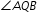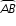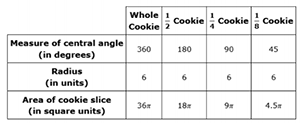# MAFS.912.G-C.2.5

Derive using similarity the fact that the length of the arc intercepted by an angle is proportional to the radius, and define the radian measure of the angle as the constant of proportionality; derive the formula for the area of a sector.
General Information
Subject Area: Mathematics
Domain-Subdomain: Geometry: Circles
Cluster: Level 3: Strategic Thinking & Complex Reasoning
Cluster: Find arc lengths and areas of sectors of circles. (Geometry - Additional Cluster) -

Clusters should not be sorted from Major to Supporting and then taught in that order. To do so would strip the coherence of the mathematical ideas and miss the opportunity to enhance the major work of the grade with the supporting clusters.

Date of Last Rating: 02/14
Status: State Board Approved
Assessed: Yes
Test Item Specifications

• Assessment Limits :
The center of dilation must be given.
• Calculator :

Neutral

• Clarification :
Students will use similarity to derive the fact that the length of the arc
intercepted by an angle is proportional to the radius, and define the
radian measure as the constant of proportionality.

Students will apply similarity to solve problems that involve the
length of the arc intercepted by an angle and the radius of a circle.

Students will derive the formula for the area of a sector.

Students will use the formula for the area of a sector to solve
problems.

• Stimulus Attributes :
Items may be set in a real-world or mathematical context
• Response Attributes :
Items may require the student to use or choose the correct unit of
measure.
Sample Test Items (2)
• Test Item #: Sample Item 1
• Question:

Circle Q has a radius,r, in units. The measure ofis xº, as shown.

A. Create an expression using r and x that can be used to find the length of, in units.

B. Then, create an expression that could be used to find the length of, in units, if circle Q were dilated by a scale factor of 3.7

• Difficulty: N/A
• Type: EE: Equation Editor

• Test Item #: Sample Item 2
• Question:

Natasha's classmates have ordered her a cookie pizza for her birthday celebration. To share it among the class, they will be slicing the cookie into equal pieces. They want everyone to have an icing packet to add to his or her slice.

In order to approximate how much icing each person will need, they want to know the area of each slice of cookie. The following table lists the radius of the cookie pizza and the area of some of the possible divisions of the cookie.This question has three parts.

Part A. What is the relationship between the area of a whole cookie with a radius of 6 units and the area of a portion whose central angle measures k degrees?

Part B. Using the information in the table, click on the blank to complete the equation for finding the area of a portion of a cookie with a radius of 6 units when given the measure of a central angle, in degrees, of the portion.

Part C. Click on the blank to complete the formula that can be used to find the area of any portion of a circle with radius w and a central angle of z degrees.

• Difficulty: N/A
• Type: : Multiple Types

## Related Courses

This benchmark is part of these courses.
1200400: Foundational Skills in Mathematics 9-12 (Specifically in versions: 2014 - 2015, 2015 - 2022 (current), 2022 and beyond)
1206310: Geometry (Specifically in versions: 2014 - 2015, 2015 - 2022 (current), 2022 and beyond)
1206320: Geometry Honors (Specifically in versions: 2014 - 2015, 2015 - 2022 (current), 2022 and beyond)
1206315: Geometry for Credit Recovery (Specifically in versions: 2014 - 2015, 2015 - 2022 (current), 2022 and beyond)
7912065: Access Geometry (Specifically in versions: 2015 - 2022 (current), 2022 and beyond)

## Related Access Points

Alternate version of this benchmark for students with significant cognitive disabilities.
MAFS.912.G-C.2.AP.5a: Find the arc length of a circle.
MAFS.912.G-C.2.AP.5b: Derive the fact that the length of the arc intercepted by an angle is proportional to the radius.
MAFS.912.G-C.2.AP.5c: Apply the formula to the area of a sector (e.g., area of a slice of pie).

## Related Resources

Vetted resources educators can use to teach the concepts and skills in this benchmark.

## Assessments

Sample 4 - High School Geometry State Interim Assessment:

This is a State Interim Assessment for 9th-12th grades.

Type: Assessment

Sample 2 - High School Geometry State Interim Assessment:

This is a State Interim Assessment for 9th-12th grade.

Type: Assessment

## Formative Assessments

Deriving the Sector Area Formula:

Students are asked to write a formula to find the area of a sector of a circle and then explain and justify that formula.

Type: Formative Assessment

Students are asked to explain why the length of an arc intercepted by an angle is proportional to the radius and then explain how that proportionality leads to a definition of the radian measure of an angle.

Type: Formative Assessment

Sector Area:

Students are asked to find the areas of sectors in two different circles.

Type: Formative Assessment

Arc Length:

Students are asked to find the lengths of arcs in two different circles.

Type: Formative Assessment

## Lesson Plans

My Favorite Slice:

The lesson introduces students to sectors of circles and illustrates ways to calculate their areas. The lesson uses pizzas to incorporate a real-world application for the of area of a sector. Students should already know the parts of a circle, how to find the circumference and area of a circle, how to find an arc length, and be familiar with ratios and percentages.

Type: Lesson Plan

Sectors of Circles:

This lesson unit is intended to help you assess how well students are able to solve problems involving area and arc length of a sector of a circle using radians. It assumes familiarity with radians and should not be treated as an introduction to the topic. This lesson is intended to help you identify and assist students who have difficulties in computing perimeters, areas, and arc lengths of sectors using formulas and finding the relationships between arc lengths, and areas of sectors after scaling.

Type: Lesson Plan

The Grass is Always Greener:

The lesson introduces area of sectors of circles then uses the areas of circles and sectors to approximate area of 2-D figures. The lesson culminates in using the area of circles and sectors of circles as spray patterns in the design of a sprinkler system between a house and the perimeter of the yard (2-D figure).

Type: Lesson Plan

Two Wheels and a Belt:

This task combines two skills: making use of the relationship between a tangent segment to a circle and the radius touching that tangent segment, and computing lengths of circular arcs given the radii and central angles.

Setting Up Sprinklers:

This modeling task involves several different types of geometric knowledge and problem-solving: finding areas of sectors of circles, using trigonometric ratios to solve right triangles, and decomposing a complicated figure involving multiple circular arcs into parts whose areas can be found.

## Text Resource

Why Tau Trumps Pi:

This informational text resource is intended to support reading in the content area. The author tries to convince the reader that two pi, or tau, occurs more often in mathematics than pi by itself. The author provides several examples and indicates the history behind society's choice of pi rather than tau.

Type: Text Resource

## Tutorial

Pi Fight: Pi vs. Tau:

Click "View Site" to open a full-screen version. This tutorial is designed to help secondary math teachers learn how to integrate literacy skills within their curriculum. This tutorial focuses on evaluating the reasoning and evidence of an argumentative claim. The focus on literacy across content areas is designed to help students independently build knowledge in different disciplines through reading and writing.

Type: Tutorial

## MFAS Formative Assessments

Arc Length:

Students are asked to find the lengths of arcs in two different circles.

Students are asked to explain why the length of an arc intercepted by an angle is proportional to the radius and then explain how that proportionality leads to a definition of the radian measure of an angle.

Deriving the Sector Area Formula:

Students are asked to write a formula to find the area of a sector of a circle and then explain and justify that formula.

Sector Area:

Students are asked to find the areas of sectors in two different circles.

## Student Resources

Vetted resources students can use to learn the concepts and skills in this benchmark.

Two Wheels and a Belt:

This task combines two skills: making use of the relationship between a tangent segment to a circle and the radius touching that tangent segment, and computing lengths of circular arcs given the radii and central angles.

Setting Up Sprinklers:

This modeling task involves several different types of geometric knowledge and problem-solving: finding areas of sectors of circles, using trigonometric ratios to solve right triangles, and decomposing a complicated figure involving multiple circular arcs into parts whose areas can be found.

## Parent Resources

Vetted resources caregivers can use to help students learn the concepts and skills in this benchmark.